﻿ Transient 2D Heat Transfer with Convection in an Anisotropic Rectangular Slab

### Transient 2D Heat Transfer with Convection in an Anisotropic Rectangular SlabOPEN ACCESSPEER-REVIEWED

## Transient 2D Heat Transfer with Convection in an Anisotropic Rectangular Slab

Mohammad Eshaghian1,, Mohammadreza Najafpour2

1Department of Computer, Payame Noor University, Tehran, Iran

2Department of Renewable Energies, Faculty of New Sciences and Technologies, University of Tehran, Tehran, Iran

### Abstract

It is not unusual that the properties of a material vary with different crystallographic orientations and this property is said to be anisotropy. Paper presented here analytically studies the transient solution to the anisotropic heat conduction in an anisotropic rectangular thin film subjected to initial arbitrary temperature function throughout the medium. An integral transformation is applied to remove the partial derivatives with respect to two spatial variables and transform the partial differential boundary value problem to ordinary differential equations. The result is the multiple integral with respect to spatial variables where the mathematics and model ling software MAPLE 18 was used to numerically calculate the summation and to plot the temperature and heat flux vector profiles.

### At a glance: Figures

1
Prev Next

• Eshaghian, Mohammad, and Mohammadreza Najafpour. "Transient 2D Heat Transfer with Convection in an Anisotropic Rectangular Slab." International Journal of Partial Differential Equations and Applications 2.3 (2014): 58-61.
• Eshaghian, M. , & Najafpour, M. (2014). Transient 2D Heat Transfer with Convection in an Anisotropic Rectangular Slab. International Journal of Partial Differential Equations and Applications, 2(3), 58-61.
• Eshaghian, Mohammad, and Mohammadreza Najafpour. "Transient 2D Heat Transfer with Convection in an Anisotropic Rectangular Slab." International Journal of Partial Differential Equations and Applications 2, no. 3 (2014): 58-61.

 Import into BibTeX Import into EndNote Import into RefMan Import into RefWorks

### 1. Introduction

Despite of a wide range of homogenous isotropic heat transfer problems which result an analytical closed form solution , most problems of anisotropic materials do not allow for a closed form solution of the heat transfer equations in this medium. Therefore, most analysis on anisotropic heattransfer is carried out numerically. Many solutions methods are proposed for the analysis of the anisotropic heat conduction. Two dimensional and steady state problems with simple geometry can be solved using infinite series method. Finite element analysis is the most popular method for solving such equations numerically using computer codes. Boundary element method is also applicable whenever the conditions on the boundaries are available, only. Buroni et al. proposed a new complexvariable formalism for the analysis of three dimensional steadystate heat transfer problems in homogeneous solids with general anisotropic behavior . Gu et al. apply the singular boundary method to steadystate heat conduction in three-dimensional anisotropic materials. They obtained that this method is accurate, convergent, stable, and computationally efficient in solving these kinds of problems . Li and Lai used the heatsource theory to develop several explicit exact solutions for heat conduction in anisotropic infinite or semi-infinite media with internal line, cylindrical-surface, or spiral-line sources . An orthotropic sphere with radiuswas considered in spherical coordinate system by Sameti and Kasaeian . The orthotropic sphere has three mutually orthogonal axes so that its mechanical properties vary along each one. A heat source at the origin of the coordinate system, generates thermal energy with constant rate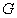at the center of the sphere and the temperature far from the sphere is. This is the case which is solved by Sameti and Kasaeianin rectangular coordinate system. They used alinear coordinate transformation is used to transform anisotropic 3D problem into the equivalent isotropic problem and the result was compared with the experimental setup in Figure 1.

### 2. Problem Description and Solution

Two dimensional transient heat conduction in an orthotropic region is considered as follows :(1)

where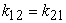is given according to reciprocity law. The heat is generated at a constant rate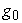. Boundaries atand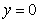are kept insulated where the heat is convected to the ambient at temperature. The orthotropic thermal conductivities in the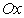and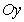directions areand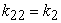, respectively. The problem can be formulated as: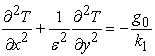(2)
Figure 1. Experimental setup used to validate analytical result using transformation technique 

whereand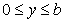. The boundary conditions are:(3)(4)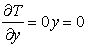(5)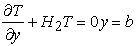(6)

where(7)

The integral transform to variable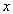is defined as: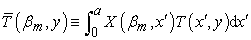(8)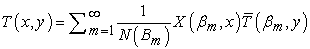(9)

where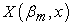and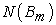can be calculated as:(10)

and values for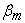are the positive roots of the following equation:(11)

The system of equations can be transformed under transformation (8) and (9) to obtain:(12)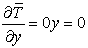(13)(14)

Solving equations (12) to (14) using typical methods for second order ordinary differential equation  yields:(15)

where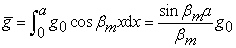(16)

Using the inverse formula in equation (9) yields: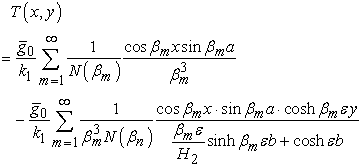(17)

The first summation in equation (17) can be expressed with: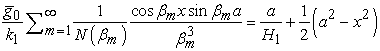(18)

### 3. Results and Discussions

Therefore, the solution in equation (18) takes the following form: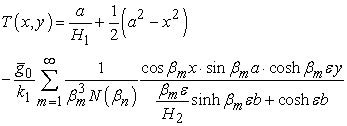(19)

Sample values for parameters in the problem are given in Table 1. The isothermal surfaces are illustrated in Figure 2 for temperature surfaces from 0 up to 80°C. If the medium was isotropic, due to the symmetric boundary conditions, the isothermal curves would be also symmetric. But because of the anisotropic nature of the square, Figure 2 is not symmetric. For example the lowest curve corresponds to the temperature 80°C and intersects the horizontal axes atwhile the vertical axes is intersected at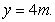The horizontal conductivity is lower which leads to the delay in heat transfer in this direction.

The compressibility of isothermal curves near in two opposite edges shows the high heat conduction rates in these zones due to the high temperature gradients. Temperature for some selective points are summarized in Table 2. Considering a point in the center of the square, the temperature reduces by 87°C walking horizontally to the right toward the point (10,5) while the temperature reduces by 113°C when going upward toward the point (5,10). The heat flux vectors are illustrated in Figure 3 where most of them have the slope more than 45° due to the anisotropicity and the differences in the convection heat transfer coefficients.

### 4. Conclusion

An analytical closed form solution was presented for a rectangular anisotropic slab subjected to convection in boundaries. An integral transformation is applied to remove the partial derivatives with respect to two spatial variables and transform the partial differential boundary value problem to ordinary second order differential equation. The solution took the form of an infinite series which is numerically solved using MAPLE. The temperature and heat flux profiles were not symmetric while compared with the isotropic medium.

### Nomenclature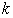: Conductivity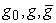: Heat generation rate: Ambient temperature: Temperature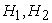: Normalized convection heat coefficients: Eigenvalues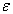: Ratio of conductivities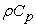: Density-specific heat product: Length

### References

  Çengel, Y. A., & Ghajar, A. J. (2014). Heat and mass transfer: fundamentals & applications. McGraw-Hill.In article  Buroni, F. C., Marczak, R. J., Denda, M., & Sáez, A. (2014). A formalism for anisotropic heat transfer phenomena: Foundations and Green’s functions. International Journal of Heat and Mass Transfer, 75, 399-409.In article CrossRef  Gu, Y., Chen, W., & He, X. Q. (2012). Singular boundary method for steady-state heat conduction in three dimensional general anisotropic media. International Journal of Heat and Mass Transfer, 55(17), 4837-4848.In article CrossRef  Li, M., & Lai, A. C. (2012). Heat-source solutions to heat conduction in anisotropic media with application to pile and borehole ground heat exchangers. Applied Energy, 96, 451-458.In article CrossRef  Sameti, M., & Kasaeian, A. (2014). Heat Diffusion in an Anisotropic Medium with Central Heat Source. International Journal, 2(2), 23-26.In article  Ozisik, M. N. (2013). Boundary value problems of heat conduction. Courier Dover Publications.In article  www.doitpoms.ac.uk/tlplib/anisotropy/thermal.php (accessed 2014/25/03).In article  Boyce, W. E., & DiPrima, R. C. (2012). Elementary differential equations and boundary value problems.In article